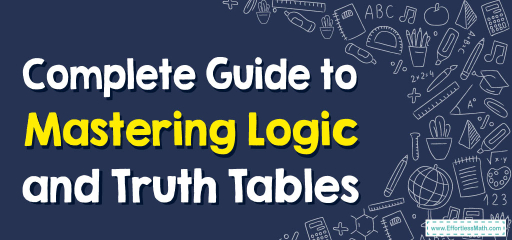# Complete Guide to Mastering Logic and Truth Tables

Welcome to the fascinating world of logic and truth tables! As you delve into the depths of geometry and mathematical reasoning, understanding the foundation of logical statements becomes crucial. Truth tables, in particular, are an invaluable tool to visualize and understand how different logical combinations play out. In this comprehensive guide, we'll walk through the basics of logic and truth tables, ensuring you grasp the core concepts with ease. So, let's dive in!## Step-by-step Guide: Logic and Truth Tables

Basic Logical Statements
Logical statements are assertions that can be either true or false, but not both. We typically use variables like $$p$$ or $$q$$ to denote these statements. For instance, $$p$$ could stand for “It is raining,” and $$q$$ could represent “I will carry an umbrella.”

Logical Operators
Logical operators are used to form compound statements from basic ones. Here are the primary logical operators:

• Conjunction (AND) denoted by $$\land$$: If both $$p$$ and $$q$$ are true, then $$p \land q$$ is true.
• Disjunction (OR) denoted by $$\lor$$: If either $$p$$ or $$q$$ is true, then $$p \lor q$$ is true.
• Negation (NOT) denoted by $$\neg$$: If $$p$$ is true, then $$\neg p$$ is false and vice versa.
• Conditional (IF…THEN) denoted by $$\rightarrow$$: “If $$p$$ then $$q$$” is false only when $$p$$ is true and $$q$$ is false.
• Biconditional (IF AND ONLY IF) denoted by $$\leftrightarrow$$: True if both $$p$$ and $$q$$ have the same truth value.

Constructing a Truth Table
A truth table lists all possible truth values for a given logical statement or compound statement. It provides a systematic way to evaluate the truth or falsity of a statement based on the truth values of its components.

Here’s how to construct one:

• List all possible combinations of truth values for the statements involved.
• Evaluate the compound statement for each combination.

### Examples

Example 1:
Construct the truth table for the logical statement $$p \lor \neg p$$:

Truth Table for $$p \lor \neg p$$
$$p$$ $$\neg p$$ $$p \lor \neg p$$
T F T
F T T

This table shows that $$p \lor \neg p$$ is always true, which makes it a tautology.

Example 2:
Construct the truth table for the conditional statement $$p \rightarrow q$$:

Truth Table for $$p \rightarrow q$$
$$p$$ $$q$$ $$p \rightarrow q$$
T T T
T F F
F T T
F F T

Note that the only time the conditional statement is false is when $$p$$ is true and $$q$$ is false.

### Practice Questions:

1. Construct the truth table for the statement $$\neg p \land q$$.
2. Evaluate the truth table for the biconditional statement $$p \leftrightarrow q$$.
3. Determine the truth value of the statement $$(p \land q) \lor \neg p$$ when $$p$$ is true and $$q$$ is false.

1. Truth Table for $$\neg p \land q$$
$$p$$ $$q$$ $$\neg p \land q$$
T T F
T F F
F T T
F F F
2. Truth Table for $$p \leftrightarrow q$$
$$p$$ $$q$$ $$p \leftrightarrow q$$
T T T
T F F
F T F
F F T
3. The truth value of $$(p \land q) \lor \neg p$$ when $$p$$ is true and $$q$$ is false is True.

### What people say about "Complete Guide to Mastering Logic and Truth Tables - Effortless Math: We Help Students Learn to LOVE Mathematics"?

No one replied yet.

X
30% OFF

Limited time only!

Save Over 30%

SAVE $5 It was$16.99 now it is \$11.99